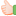1. ##Try out the answer for the following question

Between 100 and 300 how many will have digit 2 in the end, how many numbers would have digit 3 both in the end and the beginning?Reply With Quote

2. ## Re: Try out the answer for the following question

how many will have digit 2 in the end, --- 20 numbers

how many numbers would have digit 3 both in the end and the beginning? -- No number between 100 and 300.Reply With Quote

3. ##Re: Try out the answer for the following question

Using permutation for the first part we can have only 2 at the unit's place and 10 possible numbers in the ten's place.and only 1 and 2 in the hundred place thus 2 * 10 * 1=20

For the second part no such number exists between 100 and 300Reply With Quote

####Posting Permissions

• You may not post new threads
• You may not post replies
• You may not post attachments
• You may not edit your posts
•